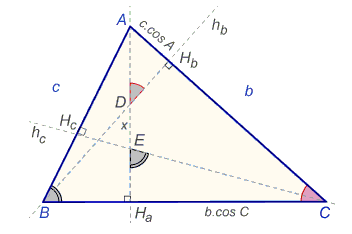# The Concurrency of the Altitudes in a Triangle A Trigonometric Proof

### Dušan Vallo February 2012

Let $ABC$ be a triangle. Using the standard notations, we denote the altitudes $h_a = AH_a$, $h_b = BH_b$, $h_c = CH_c$.

### Theorem

The three altitudes of a triangle are concurrent.

### Proof

First observe that in a right-angled triangle ABC the altitudes do meet at the right angled vertex. Now, suppose that triangle ABC is not right-angled and denote $D = h_a \cap h_b$, $E = h_a\cap h_c$. We wish to show that $D$ coincides with $E$.The triangles $ABH_a$, $ACH_a$ are right-angled and we easy derive

(1)

$h_a = c \cdot \sin B = b \cdot \sin C$.

From the right-angled triangles $ACH_a$, $ABH_b$ we obtain

(2)

$CH_a = b \cdot \cos C,$ $AH_b = c \cdot \cos A.$

The triangles $CEH_a$, $ADH_b$ are also right-angled and so $\angle ADH_b = \angle C$, $\angle CEH_a = \angle B$. From (2), we obtain

$\displaystyle AD=\frac{c\cdot \cos A}{\sin C}$, $\displaystyle EH_a=\frac{b\cdot \cos C}{\tan B}$.

Let $x=DE$. Then, for the altitude $AH_a$,

$AH_a = AD + x + EH_a$.

Using (1), this can be rewritten as

(3)

$\displaystyle c\cdot\sin C=c\cdot\frac{\cos A}{\sin C}+x+b\cdot\frac{\cos C}{\tan B}$.

Next we apply the theorem about the sum of angles in a triangle and well-known formulas

$\cos (X+Y)=\cos X\cdot\cos Y-\sin X\cdot\sin Y$,
$\cos (\pi -X)=-\cos X.$

We use the latter in (3) first with $X = A=\pi - (B+C)$ to eventually arrive at

$\displaystyle x=(b\cdot\cos C-c\cdot\cos B)\bigg[(1 - \frac{1}{\tan B\cdot\tan C}\bigg]$.

By (1), the first factor equals $b\cdot\cos C-c\cdot\cos B=h_a - h_a=0$, implying $x=0$, as required. This completes the proof.### Trigonometry